# Automata, experiments with

A method of obtaining information about the internal structure of an automaton from its behaviour as can be deduced from external experiments (that is, experiments where input words are fed into an automaton, the corresponding sequence of output words is examined and conclusions are drawn on the basis of these observations).

Experiments with automata can be used to seek approaches to the solution of the following problems:

1) Given that an automaton is in an initial state it is required to determine this state.

2) The construction of an experiment that transfers an automaton from any state to some pre-assigned state (the adjustment problem).

3) The verification of the correct functioning of an automaton. It is required to find out by means of an experiment if a given automaton functions correctly.

4) The diagnostic of an automaton. It is required to find out not only if an automaton is in good working order, but also what kind of malfunction occurs.

5) The problem of identifying an automaton from a given class (deciphering). Knowing that the automaton, viewed as a "black box" , belongs to a given set, it is required to find out which element the automaton is.

Letbe a class of initialized automata with input alphabetand output alphabet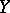. Next, let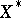be the collection of all words in, let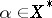and let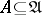. An experiment with the automatonis a set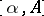of all pairs of the formsuch that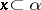and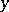is a word into whichproduces.

## The classification of experiments.

The following types of experiments are usually considered:

1) A simple unconditional experiment — a set, whereconsists of a single word. In the process of experimenting the single elementis fed into the input of the automaton and the resulting output is some word from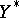.

2) A simple conditional experiment — a set, whereconsists of a single wordand where for any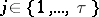the values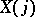depend on,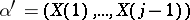. Thus, in the process of experimenting with automata every subsequent letter of the input word depends on the output word obtained earlier.

3) A multiple unconditional experiment — a generalization of a simple unconditional experiment to the case when the setis such thatconsists of more than one word. It is usually assumed that in the process of execution of the given experiment there is more than one specimen of the automaton, or that the automaton can be reset in its initial state.

4) A multiple conditional experiment — a generalization of a simple conditional experiment to the case when the setis such thatconsists of more than one word: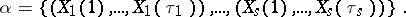Moreover, two possibilities can be distinguished here: a) for anyandthe value of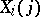depends only on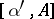, where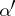consists of the single word; or b) for any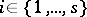and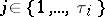the value ofdepends on, where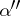is the set consisting of the collections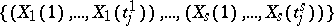and the indexforcoincides with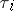if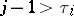or with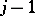if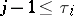.

## Measures of the complexity of experiments.

These are some numerical characteristics that estimate the degree of complexity of an experiment. For a multiple experiment the usual measures of complexity are the height of the experiment — the number of symbols of the largest input word, the multiplicity — the number of input words, and the length — the total number of symbols in all the input words. It is considered that the measure of complexity of a simple experiment is its length (the number of symbols of the input word used).

### Some results.

A Moore automatonwithstates,input andoutput symbols is called an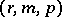-automaton. The following theorem on the distinguishability of states by a simple experiment holds: Ifis an-automaton with all states distinct, then the distinguishability of any two states can be established by means of a simple experiment of length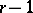, and the boundcannot be lowered (see ).

The problem of determining the initial state of an automaton can be solved by means of a simple unconditional experiment of length, where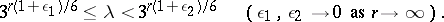Adjustment for an automaton withstates of whichare admissible can always be solved by means of a simple unconditional experiment of length, where, and this bound cannot be improved.

As a rule, the above estimates are exact only for a small number of automata. It has been established (see ) that the problem of determining the initial state for almost-all-automata with two admissible states can be solved by means of a simple unconditional experiment of length, where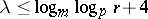, and the adjustment problem for almost-all-automata by means of a simple unconditional experiment of length, where.

There is no algorithm that would permit the decyphering of initialized Mealy automata with unknown number of states. However, it turns out that a great part of such automata can nevertheless be decyphered.

Experiments with automata are also used as tools for the control of the functioning of automata. A unified approach has been developed to problems of control of automata, which makes it possible to indicate fundamental methods for their solution. An effective algorithm has been constructed for finding all dead-end multiple experiments for various automata (see ).